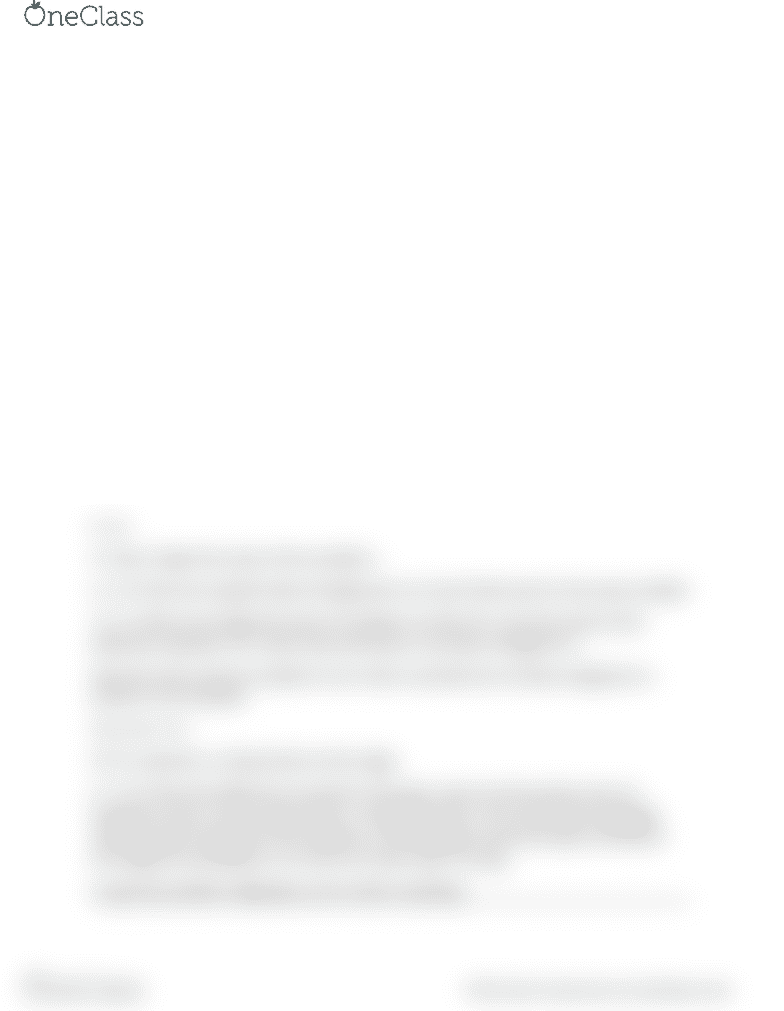# CPSC 259 Chapter 7.2: Detailed example

11 views2 pagesExample pointer code
Here is an example code that uses the ideas of reference, deference, pointer
initialization.
int a = 5; // initialize a variable with the value 5, the variable is int
int c; // initialize a variable with a garbage value
int* b; // initialize a pointer called b of type integer. So it can only refer to variable like a
b = &a; // the pointer b got referenced to variable a, so now pointer points to a
c = *b // here, I have used the deference operator *, so the variable c, gets the value of
b which is 5
Here is a more complicated example
int a, b and c // initialize three variables of type int
int* d;
int * e; //two pointers of type int created. They are bad pointers at the moment
a = 5;
b = 4;
d = &a // d gets the value of the variable a
e = d // here we using the idea of assignment so b and d both point to the same variable
*e = b //here we change the value of variable a to that of b, since the value of the
deference operator of e, which was previously a, has been changed to b
Now the most common problems occur when a pointee has not been assigned to a
pointer so for example
int a, int c = 5;
int* b; //pointer is a bad pointer at this stage
a = *b // Here the deference to pointer b has been used, but the pointer was not
assigned a value. It was a bad pointer. In languages like C, this meant that a garbage
value has been assigned to the variable a, so the program would compile correctly at
this stage but would give a run time error later when running.
In java this problem might give a error when compiling.
find more resources at oneclass.com
find more resources at oneclass.com
Unlock document

This preview shows half of the first page of the document.
Unlock all 2 pages and 3 million more documents.

Already have an account? Log in

# Get access

Grade+
\$10 USD/m
Billed \$120 USD annually
Homework Help
Class Notes
Textbook Notes
40 Verified Answers
Study Guides
1 Booster Class
Class+
\$8 USD/m
Billed \$96 USD annually
Homework Help
Class Notes
Textbook Notes
30 Verified Answers
Study Guides
1 Booster Class